xThermodynamic systemEncyclopedia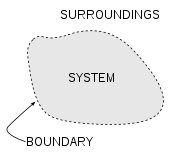A thermodynamic system is a precisely defined macroscopic
Macroscopic
The macroscopic scale is the length scale on which objects or processes are of a size which is measurable and observable by the naked eye.When applied to phenomena and abstract objects, the macroscopic scale describes existence in the world as we perceive it, often in contrast to experiences or...

region of the universe, often called a physical system
Physical system
In physics, the word system has a technical meaning, namely, it is the portion of the physical universe chosen for analysis. Everything outside the system is known as the environment, which in analysis is ignored except for its effects on the system. The cut between system and the world is a free...

, that is studied using the principles of thermodynamics
Thermodynamics
Thermodynamics is a physical science that studies the effects on material bodies, and on radiation in regions of space, of transfer of heat and of work done on or by the bodies or radiation...

.

All space in the universe outside the thermodynamic system is known as the surroundings, the environment, or a reservoir. A system is separated from its surroundings by a boundary which may be notional or real, but which by convention delimits a finite volume. Exchanges of work, heat, or matter between the system and the surroundings may take place across this boundary. Thermodynamic systems are often classified by specifying the nature of the exchanges that are allowed to occur across its boundary.

A thermodynamic system is characterized and defined by a set of thermodynamic parameters associated with the system. The parameters are experimentally measurable macroscopic properties, such as volume, pressure, temperature, electric field, and others.

The set of thermodynamic parameters necessary to uniquely define a system is called the thermodynamic state
Thermodynamic state
A thermodynamic state is a set of values of properties of a thermodynamic system that must be specified to reproduce the system. The individual parameters are known as state variables, state parameters or thermodynamic variables. Once a sufficient set of thermodynamic variables have been...

of a system. The state of a system is expressed as a functional relationship, the equation of state
Equation of state
In physics and thermodynamics, an equation of state is a relation between state variables. More specifically, an equation of state is a thermodynamic equation describing the state of matter under a given set of physical conditions...

, between its parameters. A system is in thermodynamic equilibrium
Thermodynamic equilibrium
In thermodynamics, a thermodynamic system is said to be in thermodynamic equilibrium when it is in thermal equilibrium, mechanical equilibrium, radiative equilibrium, and chemical equilibrium. The word equilibrium means a state of balance...

when the state of the system does not change with time.

Nicolas Léonard Sadi Carnot was a French military engineer who, in his 1824 Reflections on the Motive Power of Fire, gave the first successful theoretical account of heat engines, now known as the Carnot cycle, thereby laying the foundations of the second law of thermodynamics...

described a thermodynamic system as the working substance under study.

## Overview

Thermodynamics describes the physics of matter using the concept of the thermodynamic system, a region of the universe that is under study. All quantities, such as pressure
Pressure
Pressure is the force per unit area applied in a direction perpendicular to the surface of an object. Gauge pressure is the pressure relative to the local atmospheric or ambient pressure.- Definition :...

or mechanical work
Mechanical work
In physics, work is a scalar quantity that can be described as the product of a force times the distance through which it acts, and it is called the work of the force. Only the component of a force in the direction of the movement of its point of application does work...

, in an equation refer to the system unless labeled otherwise. As thermodynamics is fundamentally concerned with the flow and balance of energy and matter, systems are distinguished depending on the kinds of interaction they undergo and the types of energy
Energy
In physics, energy is an indirectly observed quantity. It is often understood as the ability a physical system has to do work on other physical systems...

they exchange with the surrounding environment.

Isolated systems are completely isolated from their environment. They do not exchange heat, work or matter with their environment. An example of an isolated system is a completely insulated rigid container, such as a completely insulated gas cylinder. Closed systems are able to exchange energy (heat and work) but not matter with their environment. A greenhouse is an example of a closed system exchanging heat but not work with its environment. Whether a system exchanges heat, work or both is usually thought of as a property of its boundary. Open systems may exchange any form of energy as well as matter with their environment. A boundary allowing matter exchange is called permeable. The ocean would be an example of an open system.

In practice, a system can never be absolutely isolated from its environment, because there is always at least some slight coupling, such as gravitational attraction. In analyzing a system in steady-state, the energy into the system is equal to the energy leaving the system http://www.tpub.com/content/doe/h1012v1/css/h1012v1_94.htm.

An example system is the system of hot liquid water and solid table salt
Sodium chloride
Sodium chloride, also known as salt, common salt, table salt or halite, is an inorganic compound with the formula NaCl. Sodium chloride is the salt most responsible for the salinity of the ocean and of the extracellular fluid of many multicellular organisms...

in a sealed, insulated test tube held in a vacuum (the surroundings). The test tube constantly loses heat in the form of black-body radiation, but the heat loss progresses very slowly. If there is another process going on in the test tube, for example the dissolution
Solvation
Solvation, also sometimes called dissolution, is the process of attraction and association of molecules of a solvent with molecules or ions of a solute...

of the salt crystal
Crystal
A crystal or crystalline solid is a solid material whose constituent atoms, molecules, or ions are arranged in an orderly repeating pattern extending in all three spatial dimensions. The scientific study of crystals and crystal formation is known as crystallography...

s, it will probably occur so quickly that any heat lost to the test tube during that time can be neglected. Thermodynamics in general does not measure time, but it does sometimes accept limitations on the time frame of a process.

## History

The first to develop the concept of a thermodynamic system was the French physicist Sadi Carnot
Nicolas Léonard Sadi Carnot was a French military engineer who, in his 1824 Reflections on the Motive Power of Fire, gave the first successful theoretical account of heat engines, now known as the Carnot cycle, thereby laying the foundations of the second law of thermodynamics...

whose 1824 Reflections on the Motive Power of Fire
Reflections on the Motive Power of Fire
In 1824, French physicist Sadi Carnot published the book Reflections on the Motive Power of Fire and on Machines Fitted to Develop that Power....

studied what he called the working substance, e.g., typically a body of water vapor, in steam engine
Steam engine
A steam engine is a heat engine that performs mechanical work using steam as its working fluid.Steam engines are external combustion engines, where the working fluid is separate from the combustion products. Non-combustion heat sources such as solar power, nuclear power or geothermal energy may be...

s, in regards to the system's ability to do work when heat is applied to it. The working substance could be put in contact with either a heat reservoir (a boiler), a cold reservoir (a stream of cold water), or a piston (to which the working body could do work by pushing on it). In 1850, the German physicist Rudolf Clausius
Rudolf Clausius
Rudolf Julius Emanuel Clausius , was a German physicist and mathematician and is considered one of the central founders of the science of thermodynamics. By his restatement of Sadi Carnot's principle known as the Carnot cycle, he put the theory of heat on a truer and sounder basis...

generalized this picture to include the concept of the surroundings, and began referring to the system as a "working body." In his 1850 manuscript On the Motive Power of Fire, Clausius wrote:
The article Carnot heat engine
Carnot heat engine
A Carnot heat engine is a hypothetical engine that operates on the reversible Carnot cycle. The basic model for this engine was developed by Nicolas Léonard Sadi Carnot in 1824...

shows the original piston-and-cylinder diagram used by Carnot in discussing his ideal engine; below, we see the Carnot engine as is typically modeled in current use: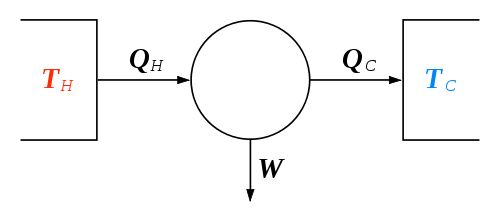In the diagram shown, the "working body" (system), a term introduced by Clausius in 1850, can be any fluid or vapor body through which heat
Heat
In physics and thermodynamics, heat is energy transferred from one body, region, or thermodynamic system to another due to thermal contact or thermal radiation when the systems are at different temperatures. It is often described as one of the fundamental processes of energy transfer between...

Q can be introduced or transmitted through to produce work
Mechanical work
In physics, work is a scalar quantity that can be described as the product of a force times the distance through which it acts, and it is called the work of the force. Only the component of a force in the direction of the movement of its point of application does work...

. In 1824, Sadi Carnot, in his famous paper Reflections on the Motive Power of Fire, had postulated that the fluid body could be any substance capable of expansion, such as vapor of water, vapor of alcohol, vapor of mercury, a permanent gas, or air, etc. Although, in these early years, engines came in a number of configurations, typically QH was supplied by a boiler, wherein water was boiled over a furnace; QC was typically a stream of cold flowing water in the form of a condenser
Condenser
Condenser may refer to:*Condenser , a device or unit used to condense vapor into liquid. More specific articles on some types include:*Air coil used in HVAC refrigeration systems...

located on a separate part of the engine. The output work W here is the movement of the piston as it is used to turn a crank-arm, which was then typically used to turn a pulley so to lift water out of flooded salt mines. Carnot defined work as "weight lifted through a height."

## Boundary

A system boundary is a real or imaginary two-dimensional closed surface that encloses or demarcates the volume or region that a thermodynamic system occupies, across which quantities such as heat
Heat
In physics and thermodynamics, heat is energy transferred from one body, region, or thermodynamic system to another due to thermal contact or thermal radiation when the systems are at different temperatures. It is often described as one of the fundamental processes of energy transfer between...

, mass
Mass
Mass can be defined as a quantitive measure of the resistance an object has to change in its velocity.In physics, mass commonly refers to any of the following three properties of matter, which have been shown experimentally to be equivalent:...

, or work
Work (thermodynamics)
In thermodynamics, work performed by a system is the energy transferred to another system that is measured by the external generalized mechanical constraints on the system. As such, thermodynamic work is a generalization of the concept of mechanical work in mechanics. Thermodynamic work encompasses...

can flow. In short, a thermodynamic boundary is a geometrical division between a system and its surroundings. Topologically
Topology
Topology is a major area of mathematics concerned with properties that are preserved under continuous deformations of objects, such as deformations that involve stretching, but no tearing or gluing...

, it is usually considered to be nearly or piecewise smoothly homeomorphic
Homeomorphism
In the mathematical field of topology, a homeomorphism or topological isomorphism or bicontinuous function is a continuous function between topological spaces that has a continuous inverse function. Homeomorphisms are the isomorphisms in the category of topological spaces—that is, they are...

with a two-sphere, because a system is usually considered to be simply connected
Simply connected space
In topology, a topological space is called simply connected if it is path-connected and every path between two points can be continuously transformed, staying within the space, into any other path while preserving the two endpoints in question .If a space is not simply connected, it is convenient...

.

Boundaries can also be fixed (e.g. a constant volume reactor) or moveable (e.g. a piston). For example, in an engine, a fixed boundary means the piston is locked at its position; as such, a constant volume process occurs. In that same engine, a moveable boundary allows the piston to move in and out. Boundaries may be real or imaginary. For closed systems, boundaries are real while for open system boundaries are often imaginary. For theoretical purposes, a boundary may be declared to be adiabatic, isothermal, diathermal, insulating, permeable, or semipermeable, but actual physical materials that provide such idealized properties are not always readily available.

Anything that passes across the boundary that effects a change in the internal energy needs to be accounted for in the energy balance equation. The volume can be the region surrounding a single atom resonating energy, such as Max Planck
Max Planck
Max Karl Ernst Ludwig Planck, ForMemRS, was a German physicist who actualized the quantum physics, initiating a revolution in natural science and philosophy. He is regarded as the founder of the quantum theory, for which he received the Nobel Prize in Physics in 1918.-Life and career:Planck came...

defined in 1900; it can be a body of steam or air in a steam engine
Steam engine
A steam engine is a heat engine that performs mechanical work using steam as its working fluid.Steam engines are external combustion engines, where the working fluid is separate from the combustion products. Non-combustion heat sources such as solar power, nuclear power or geothermal energy may be...

Sadi Carnot may refer to:*Nicolas Léonard Sadi Carnot , French physicist*Marie François Sadi Carnot , president of the third French Republic, and nephew of Nicolas Léonard Sadi Carnot...

defined in 1824; it can be the body of a tropical cyclone
Cyclone
In meteorology, a cyclone is an area of closed, circular fluid motion rotating in the same direction as the Earth. This is usually characterized by inward spiraling winds that rotate anticlockwise in the Northern Hemisphere and clockwise in the Southern Hemisphere of the Earth. Most large-scale...

, such as Kerry Emanuel
Kerry Emanuel
Kerry Emanuel is an American professor of meteorology currently working at the Massachusetts Institute of Technology in Cambridge. In particular he has specialized in atmospheric convection and the mechanisms acting to intensify hurricanes. He coined the term "hypercane" in 1994. In 2007, he was...

theorized in 1986 in the field of atmospheric thermodynamics
Atmospheric thermodynamics
Atmospheric thermodynamics is the study of heat to work transformations in the earth’s atmospheric system in relation to weather or climate...

; it could also be just one nuclide (i.e. a system of quarks) as hypothesized in quantum thermodynamics
Quantum thermodynamics
In the physical sciences, quantum thermodynamics is the study of heat and work dynamics in quantum systems. Approximately, quantum thermodynamics attempts to combine thermodynamics and quantum mechanics into a coherent whole. The essential point at which "quantum mechanics" began was when, in...

.

## Surroundings

The system is the part of the universe being studied, while the surroundings is the remainder of the universe that lies outside the boundaries of the system. It is also known as the environment, and the reservoir. Depending on the type of system, it may interact with the system by exchanging mass, energy (including heat and work), momentum
Momentum
In classical mechanics, linear momentum or translational momentum is the product of the mass and velocity of an object...

, electric charge
Electric charge
Electric charge is a physical property of matter that causes it to experience a force when near other electrically charged matter. Electric charge comes in two types, called positive and negative. Two positively charged substances, or objects, experience a mutual repulsive force, as do two...

, or other conserved properties
Conservation law
In physics, a conservation law states that a particular measurable property of an isolated physical system does not change as the system evolves....

. The environment is ignored in analysis of the system, except in regards to these interactions.

## Open systemIn open systems, matter may flow in and out of the system boundaries. The first law of thermodynamics for open systems states: the increase in the internal energy of a system is equal to the amount of energy added to the system by matter flowing in and by heating, minus the amount lost by matter flowing out and in the form of work done by the system. The first law for open systems is given by: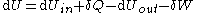where Uin is the average internal energy
Internal energy
In thermodynamics, the internal energy is the total energy contained by a thermodynamic system. It is the energy needed to create the system, but excludes the energy to displace the system's surroundings, any energy associated with a move as a whole, or due to external force fields. Internal...

entering the system and Uout is the average internal energy leaving the system

The region of space enclosed by open system boundaries is usually called a control volume
Control volume
In fluid mechanics and thermodynamics, a control volume is a mathematical abstraction employed in the process of creating mathematical models of physical processes. In an inertial frame of reference, it is a volume fixed in space or moving with constant velocity through which the fluid flows...

, and it may or may not correspond to physical walls. If we choose the shape of the control volume such that all flow in or out occurs perpendicular to its surface, then the flow of matter into the system performs work as if it were a piston of fluid pushing mass into the system, and the system performs work on the flow of matter out as if it were driving a piston of fluid. There are then two types of work performed: flow work described above which is performed on the fluid (this is also often called PV work) and shaft work which may be performed on some mechanical device. These two types of work are expressed in the equation: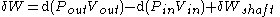Substitution into the equation above for the control volume cv yields: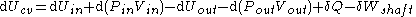The definition of enthalpy
Enthalpy
Enthalpy is a measure of the total energy of a thermodynamic system. It includes the internal energy, which is the energy required to create a system, and the amount of energy required to make room for it by displacing its environment and establishing its volume and pressure.Enthalpy is a...

, H, permits us to use this thermodynamic potential to account for both internal energy and PV work in fluids for open systems: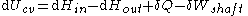A unit operation is considered to be at a steady state with respect to an operation variable if that variable does not change with time. Such a process is called a steady-state process.-Example:...

operation of a device (see turbine
Turbine
A turbine is a rotary engine that extracts energy from a fluid flow and converts it into useful work.The simplest turbines have one moving part, a rotor assembly, which is a shaft or drum with blades attached. Moving fluid acts on the blades, or the blades react to the flow, so that they move and...

, pump
Pump
A pump is a device used to move fluids, such as liquids, gases or slurries.A pump displaces a volume by physical or mechanical action. Pumps fall into three major groups: direct lift, displacement, and gravity pumps...

, and engine
Engine
An engine or motor is a machine designed to convert energy into useful mechanical motion. Heat engines, including internal combustion engines and external combustion engines burn a fuel to create heat which is then used to create motion...

), any system property within the control volume is independent of time. Therefore, the internal energy of the system enclosed by the control volume remains constant, which implies that dUcv in the expression above may be set equal to zero. This yields a useful expression for the power
Power (physics)
In physics, power is the rate at which energy is transferred, used, or transformed. For example, the rate at which a light bulb transforms electrical energy into heat and light is measured in watts—the more wattage, the more power, or equivalently the more electrical energy is used per unit...

generation or requirement for these devices in the absence of chemical reaction
Chemical reaction
A chemical reaction is a process that leads to the transformation of one set of chemical substances to another. Chemical reactions can be either spontaneous, requiring no input of energy, or non-spontaneous, typically following the input of some type of energy, such as heat, light or electricity...

s: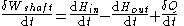This expression is described by the diagram above.

## Closed system

In a closed system, no mass may be transferred in or out of the system boundaries. The system will always contain the same amount of matter, but heat and work can be exchanged across the boundary of the system. Whether a system can exchange heat, work, or both is dependent on the property of its boundary.
• Adiabatic boundary – not allowing any heat exchange
• Rigid boundary – not allowing exchange of work

One example is fluid being compressed by a piston in a cylinder. Another example of a closed system is a bomb calorimeter, a type of constant-volume calorimeter used in measuring the heat of combustion of a particular reaction. Electrical energy travels across the boundary to produce a spark between the electrodes and initiates combustion. Heat transfer occurs across the boundary after combustion but no mass transfer takes place either way.

Beginning with the first law of thermodynamics for an open system, this is expressed as: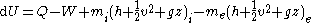where U is internal energy, Q is heat transfer, W is work, and since no mass is transferred in or out of the system, both expressions involving mass flow, , zeroes, and the first law of thermodynamics for a closed system is derived. The first law of thermodynamics for a closed system states that the amount of internal energy within the system equals the difference between the amount of heat added to or extracted from the system and the work done by or to the system. The first law for closed systems is stated by: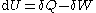where U is the average internal energy within the system, Q is the heat added to or extracted from the system and W is the work done by or to the system.

Substituting the amount of work needed to accomplish a reversible process, which is stated by: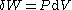where P is the measured pressure and V is the volume, and the heat required to accomplish a reversible process stated by the second law of thermodynamics, the universal principle of entropy, stated by: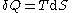where T is the absolute temperature and S is the entropy of the system, derives the fundamental thermodynamic relationship used to compute changes in internal energy, which is expressed as: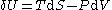For a simple system, with only one type of particle (atom or molecule), a closed system amounts to a constant number of particles. However, for systems which are undergoing a chemical reaction
Chemical equilibrium
In a chemical reaction, chemical equilibrium is the state in which the concentrations of the reactants and products have not yet changed with time. It occurs only in reversible reactions, and not in irreversible reactions. Usually, this state results when the forward reaction proceeds at the same...

, there may be all sorts of molecules being generated and destroyed by the reaction process. In this case, the fact that the system is closed is expressed by stating that the total number of each elemental atom is conserved, no matter what kind of molecule it may be a part of. Mathematically: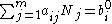where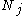is the number of j-type molecules,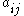is the number of atoms of element i in molecule j and bi0 is the total number of atoms of element i in the system, which remains constant, since the system is closed. There will be one such equation for each different element in the system.

## Isolated system

An isolated system is more restrictive than a closed system as it does not interact with its surroundings in any way. Mass and energy remains constant within the system, and no energy or mass transfer takes place across the boundary. As time passes in an isolated system, internal differences in the system tend to even out and pressures and temperatures tend to equalize, as do density differences. A system in which all equalizing processes have gone practically to completion is considered to be in a state of thermodynamic equilibrium
Thermodynamic equilibrium
In thermodynamics, a thermodynamic system is said to be in thermodynamic equilibrium when it is in thermal equilibrium, mechanical equilibrium, radiative equilibrium, and chemical equilibrium. The word equilibrium means a state of balance...

.

Truly isolated physical systems do not exist in reality (except perhaps for the universe as a whole), because, for example, there is always gravity between a system with mass and masses elsewhere. However, real systems may behave nearly as an isolated system for finite (possibly very long) times. The concept of an isolated system can serve as a useful model approximating many real-world situations. It is an acceptable idealization
Idealization
Idealization is the process by which scientific models assume facts about the phenomenon being modeled that are strictly false. Often these assumptions are used to make models easier to understand or solve. Many times idealizations do not harm the predictive accuracy of the model for one reason or...

used in constructing mathematical model
Mathematical model
A mathematical model is a description of a system using mathematical concepts and language. The process of developing a mathematical model is termed mathematical modeling. Mathematical models are used not only in the natural sciences and engineering disciplines A mathematical model is a...

s of certain natural phenomena
Phenomenon
A phenomenon , plural phenomena, is any observable occurrence. Phenomena are often, but not always, understood as 'appearances' or 'experiences'...

.

In the attempt to justify the postulate of entropy
Entropy
Entropy is a thermodynamic property that can be used to determine the energy available for useful work in a thermodynamic process, such as in energy conversion devices, engines, or machines. Such devices can only be driven by convertible energy, and have a theoretical maximum efficiency when...

increase in the second law of thermodynamics
Second law of thermodynamics
The second law of thermodynamics is an expression of the tendency that over time, differences in temperature, pressure, and chemical potential equilibrate in an isolated physical system. From the state of thermodynamic equilibrium, the law deduced the principle of the increase of entropy and...

, Boltzmann’s H-theorem
H-theorem
In Classical Statistical Mechanics, the H-theorem, introduced by Ludwig Boltzmann in 1872, describes the increase in the entropy of an ideal gas in an irreversible process. H-theorem follows from considerations of Boltzmann's equation...

used equations which assumed a system (for example, a gas
Gas
Gas is one of the three classical states of matter . Near absolute zero, a substance exists as a solid. As heat is added to this substance it melts into a liquid at its melting point , boils into a gas at its boiling point, and if heated high enough would enter a plasma state in which the electrons...

) was isolated. That is all the mechanical degrees of freedom could be specified, treating the walls simply as mirror
Mirror
A mirror is an object that reflects light or sound in a way that preserves much of its original quality prior to its contact with the mirror. Some mirrors also filter out some wavelengths, while preserving other wavelengths in the reflection...

boundary conditions. This inevitably led to Loschmidt's paradox
Loschmidt's paradox, also known as the reversibility paradox, is the objection that it should not be possible to deduce an irreversible process from time-symmetric dynamics...

. However, if the stochastic
Stochastic
Stochastic refers to systems whose behaviour is intrinsically non-deterministic. A stochastic process is one whose behavior is non-deterministic, in that a system's subsequent state is determined both by the process's predictable actions and by a random element. However, according to M. Kac and E...

behavior of the molecule
Molecule
A molecule is an electrically neutral group of at least two atoms held together by covalent chemical bonds. Molecules are distinguished from ions by their electrical charge...

s in actual walls is considered, along with the randomizing effect of the ambient, background thermal radiation
Thermal radiation is electromagnetic radiation generated by the thermal motion of charged particles in matter. All matter with a temperature greater than absolute zero emits thermal radiation....

, Boltzmann’s assumption of molecular chaos
Molecular chaos
In kinetic theory in physics, molecular chaos is the assumption that the velocities of colliding particles are uncorrelated, and independent of position...

can be justified.

The second law of thermodynamics is only true for isolated systems. It states that the entropy of an isolated system not in equilibrium will tend to increase over time, approaching maximum value at equilibrium. Overall, in an isolated system, the available energy can never increase, and it complement, entropy, can never decrease. A closed system's entropy can decrease.

It is important to note that isolated systems are not equivalent to closed systems. Closed systems cannot exchange matter with the surroundings, but can exchange energy. Isolated systems can exchange neither matter nor energy with their surroundings, and as such are only theoretical and do not exist in reality (except, possibly, the entire universe).

It is worth noting that 'closed system' is often used in thermodynamics discussions when 'isolated system' would be correct - i.e. there is an assumption that energy does not enter or leave the system.

## Systems in equilibrium

At thermodynamic equilibrium
Thermodynamic equilibrium
In thermodynamics, a thermodynamic system is said to be in thermodynamic equilibrium when it is in thermal equilibrium, mechanical equilibrium, radiative equilibrium, and chemical equilibrium. The word equilibrium means a state of balance...

, a system's properties are, by definition, unchanging in time. Systems in equilibrium are much simpler and easier to understand than systems which are not in equilibrium. Often, when analyzing a thermodynamic process, it can be assumed that each intermediate state in the process is at equilibrium. This will also considerably simplify the analysis.

In isolated systems it is consistently observed that as time goes on internal rearrangements diminish and stable conditions are approached. Pressures and temperatures tend to equalize, and matter arranges itself into one or a few relatively homogeneous phase
Phase (matter)
In the physical sciences, a phase is a region of space , throughout which all physical properties of a material are essentially uniform. Examples of physical properties include density, index of refraction, and chemical composition...

s. A system in which all processes of change have gone practically to completion is considered to be in a state of thermodynamic equilibrium
Thermodynamic equilibrium
In thermodynamics, a thermodynamic system is said to be in thermodynamic equilibrium when it is in thermal equilibrium, mechanical equilibrium, radiative equilibrium, and chemical equilibrium. The word equilibrium means a state of balance...

. The thermodynamic properties of a system in equilibrium are unchanging in time. Equilibrium system states are much easier to describe in a deterministic manner than non-equilibrium states.

In thermodynamic processes, large departures from equilibrium during intermediate steps are associated with increases in entropy and increases in the production of heat rather than useful work. It can be shown that for a process to be reversible
Reversible process (thermodynamics)
In thermodynamics, a reversible process, or reversible cycle if the process is cyclic, is a process that can be "reversed" by means of infinitesimal changes in some property of the system without loss or dissipation of energy. Due to these infinitesimal changes, the system is in thermodynamic...

, each step in the process must be reversible. For a step in a process to be reversible, the system must be in equilibrium throughout the step. That ideal cannot be accomplished in practice because no step can be taken without perturbing the system from equilibrium, but the ideal can be approached by making changes slowly.